﻿ A Short Note on Converting a Series into a Form of FractionApplied Mathematics

p-ISSN: 2163-1409    e-ISSN: 2163-1425

2021;  11(1): 12-14

doi:10.5923/j.am.20211101.03

Received: Apr. 8, 2021; Accepted: Apr. 24, 2021; Published: Apr. 26, 2021### A Short Note on Converting a Series into a Form of Fraction

Ameha Tefera Tessema

Strategic Planning, Commercial Bank of Ethiopia, Addis Ababa, Ethiopia

Correspondence to: Ameha Tefera Tessema, Strategic Planning, Commercial Bank of Ethiopia, Addis Ababa, Ethiopia.
 Email: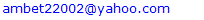Abstract

Let for all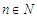and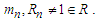If the sum of a series defined as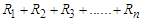and if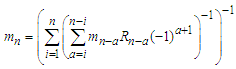where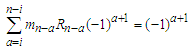for n=i,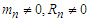,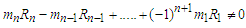then the sum of a series can be converted into fraction as follows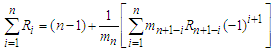.

Keywords: Fractional series, Continued fraction, Series

Cite this paper: Ameha Tefera Tessema, A Short Note on Converting a Series into a Form of Fraction, Applied Mathematics, Vol. 11 No. 1, 2021, pp. 12-14. doi: 10.5923/j.am.20211101.03.

1. Introduction
2. Conclusions

### 1. Introduction

Continued fraction can be defined as dividing the numerator by successive real numbers. Continues fraction algorism is a general factoring method which has received a great deal of attention in recent years and in order to implement it on a highly parallel computer, like the massively parallel processor, it is necessary to be able to compute certain numbers which occur at widely-spaced intervals within the continued fraction expansion of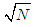where N is the number to be factored . The Massively Parallel Processor (MPP) is a highly parallel scientific computer which was originally intended for image processing and analysis applications but it is also suitable for a large range of other scientific applications . Continued fractions are used in analysing Frieze patterns , in R_dseth's formula for Frobenius numbers , in computing the Jacobi symbol , in stream ciphers , in pseudo-random number generators , in cryptanalysis of the RSA public-key cryptosystem with small private exponents . There are numerous beautiful continued fractions developed by Euler, Lagrange, Gauss, Stieltjes, Ramanujan and so on using various methods. However, according to this paper the sum of real numbers can be written as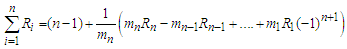where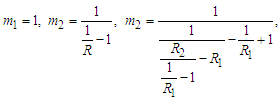and so on.
Still nowadays there is no any stated general formula which serves to transform every kind of series into a form of fraction since the formulas existed function valid only for specific values of rational integers. However, on this paper I want to show that how every kind of series can be converted into a form of fraction. Transforming a series into a form of fraction will help to solve various mathematical problems since any series can be converted into fractional series or rational fraction series. Since rational function, which can be defined by rational fraction, can be used in the series, converting a series into form of fraction can be used to approximate or model more complex equations in science and engineering including fields and forces in physics, spectroscopy in analytical chemistry, enzyme kinetics in biochemistry, electronic circuitry, aerodynamics, medicine concentrations in vivo, wave functions for atoms and molecules, optics and photography to improve image resolution, and acoustics and sound .
Theorem 1: Let for all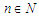and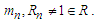If the sum of a series defined as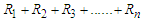and if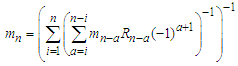where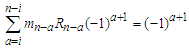for n=i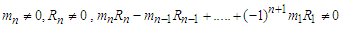then the sum of a series can be converted into fraction as follows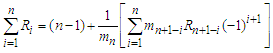Proof. Suppose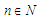and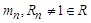such that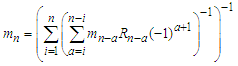where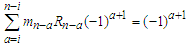for n=i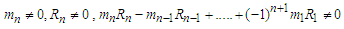and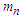can be calculated as follows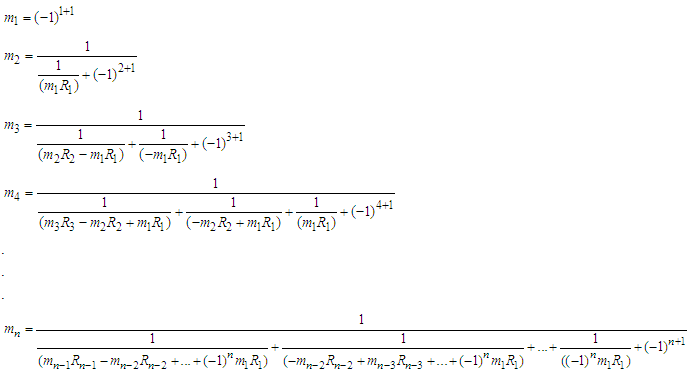The sum of real numbers can be represented as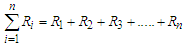which can also calculate as follows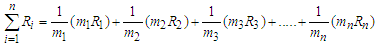In order to get cancellation one, split the terms in brackets in the following form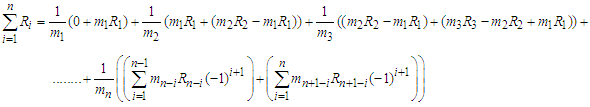Collecting like terms, we have the following series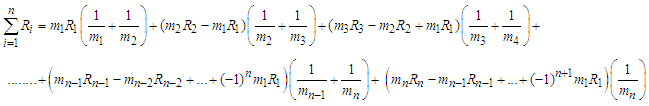And putting the value of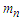in the above series, we have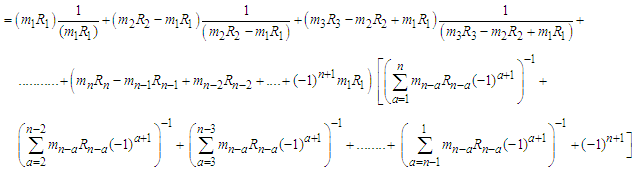Thus we have a series converted in the form of fraction as follow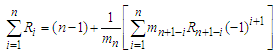### 2. Conclusions

Since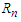is arbitrary real number, the sum of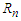can be represented as a form of fraction. The difference between consecutive fractional terms,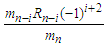where i=0,1,2,…., diminishes without limit for each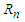is divided by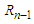up to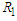and since the form of fraction are finite in number, the fraction is said to be definite. Therefor the formula developed by this paper serves as a general formula to convert every kind of series into a form of fraction.

### References

  A. M. Kane, \On the use of continued fractions for stream ciphers," in Proceedings of the 2009 International Conference on Security & Management, 2009, pp. 583-589.  A. V. Ustinov, \Geometric proof of R_dseth's formula for Frobenius numbers," Proceedings of the Steklov Institute of Mathematics, vol. 276, pp. 275-282, 2012.  D. Boneh and G. Durfee, \Cryptanalysis of RSA with private key d less than N0:292," IEEE Transactions on Information Theory, vol. 46, pp. 1339-1349, 2000.  E. Bach and J. Shallit, Algorithmic number theory, Volume I: E_cient algorithms. MIT Press, 1996.  H. S. M. Coxeter, \Frieze patterns," Acta Arithmetica, vol. 18, pp. 297-310, 1971.  J. L. Potter, The Massively Parallel Processor, MIT Press (1985). Google Scholar.  L. Blum, M. Blum, and M. Shub, \A simple unpredictable pseudo-random number generator," SIAM Journal on Computing, vol. 15, no. 2, pp. 364-383, 1986.  Williams, H.C. and Wundertich M.C. (1987). On The Parallel Generation of the Residues for the Continued fraction Factoring Algorithm. Mathematics of Computation Journal, Volume 48, Number 177, the American mathematical Society press, U.S.A. PP.405-423.  https://en.wikipedia.org/wiki/Rational_function